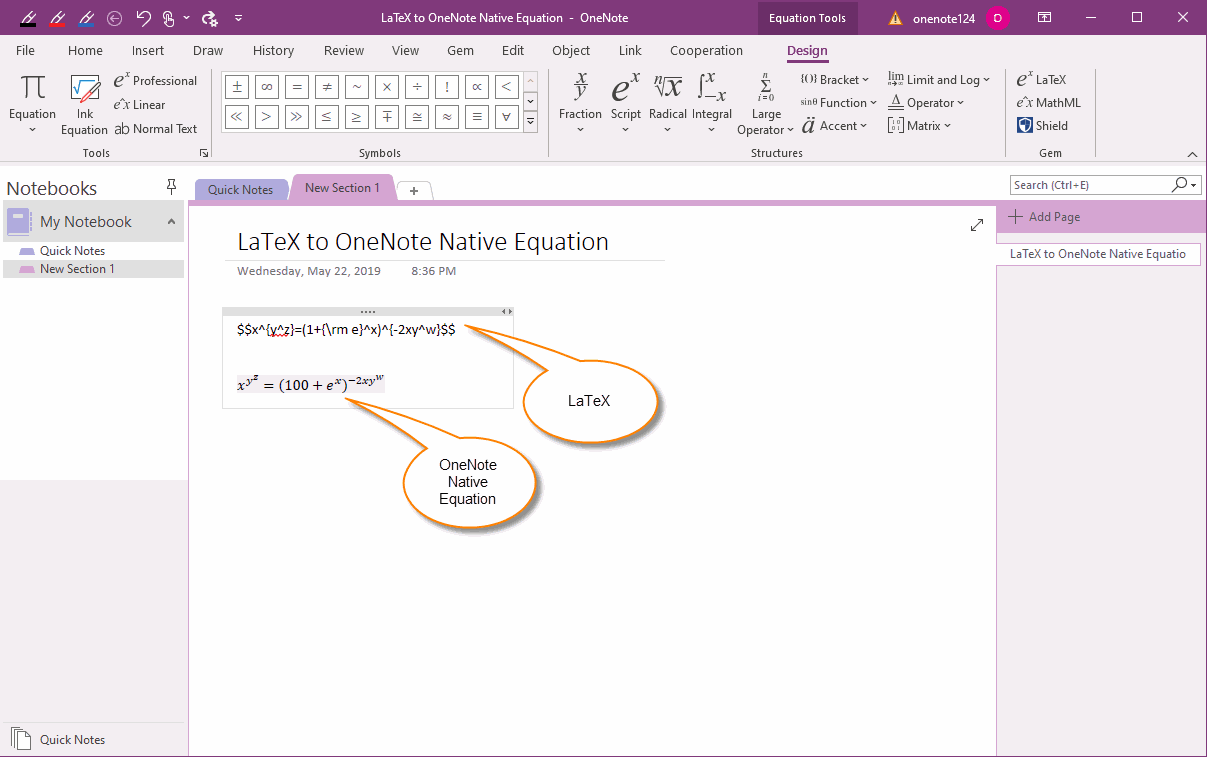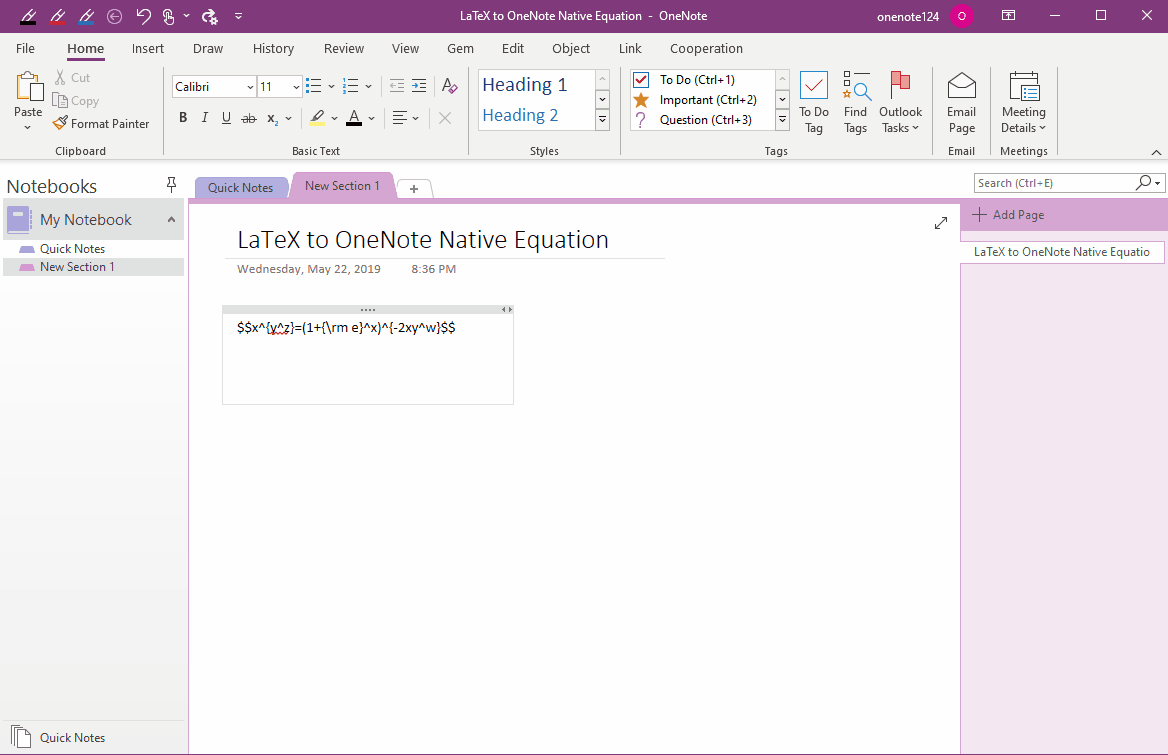### EXE: Convert LaTeX Copied from Web Page to OneNote Native Equation

There are many LaTeX expressions in the web pages, and they are not easy to generate to equation quickly in OneNote.
Gem for OneNote add-in now offers a "LaTeX Equation" feature that allows you to copy LaTeX expressions from web pages, convert them into OneNote as native equations.

## Convert LaTeX from Webpage to OneNote's Native Equation

Steps:
1. In OneNote “Insert” tab -> click “Equation”. (Or press keyboard shortcut:  Alt+=)
2. New tab “Equation Tools/Design” will appears in OneNote ribbon.
3. Click the Gem’s feature “LaTeX” end of Design tab.
4. Copy a LaTeX expression from webpage. For example: $$x^{y^z}=(1+{\rm e}^x)^{-2xy^w}$$
5. Paste to LaTeX input box. On the right Preview pane, it will display the correct equation.
6. Finally, press “OK”. Gem save it to OneNote as native equation.## OneNote Native Equation

OneNote's native equation can be modified directly in OneNote without need additional tools.

You can see the OneNote equation generated by Gem's LaTeX feature, which can be modified directly in OneNote.## Demonstration